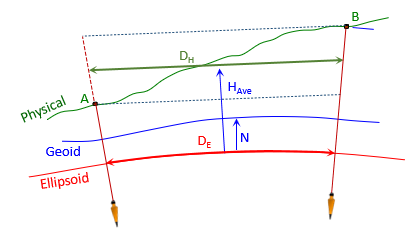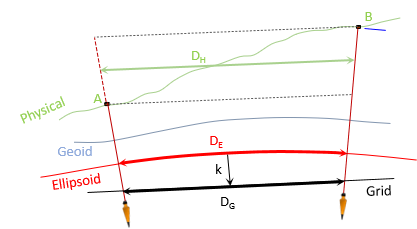### 2. Ground Distance to Grid

#### a. Ground to Ellipsoid

Going from ground to the ellipsoid is independent of the grid system.Figure K-2 Ground to Geodetic

Use Equation H-2 to reduce ground to the ellipsoidEquation H-2
Te equation uses a line's average elevation and the average geoid height to determine the geodetic length on the ellipsoid.
RE is 20.906 x 106 ft.

Jerry's geoid height is -33.902 meters computed using the GEOID18 model. It is negative because the geoid is below the ellipsoid in Wisconsin.
Since we're working in feet, the geoid height must be converted from meters:Geoid height generally does not vary significantly over an area of this size. Since Jerry is centrally located, -111.2 ft can be used as a project average.

##### (1) Geodetic distance Jerry-A1##### (2) Geodetic distance Jerry-B7#### b. Ellipsoid to GridFigure K-3 Geodetic to Grid

To go from ellipsoid to grid, the geodetic distance multiplied by the grid scale factor, k, Equation H-3.Equation H-3

The grid scale in Equation H-3 can be:

Jerry's scale used for the entire project
Average of the endpoint scales for each line
A weighted average scale, Equation H-4, for each line based on scales at the mid- and endpointsEquation H-4

We'll compute the grid distance each way and compare their results.

##### (1) Using Jerry's scale: k=0.99996 957.
 Line Grid Distance Jerry-A1:Jerry-B7:##### (2) Average scale for each line

Using software, scale at points A1 and B7 can be determined using their approximate coordinates. NGS's NCAT can be used for this as well as the NAD 83 Coordinate Conversion workbook.

 Point Scale A1 0.99996 8421 B7 0.99996 8894

Multiplying each line's geodetic distance by its average scale:

 Line Average k Grid Dist Jerry-A1 0.99996 8996 4281.768 Jerry-B7 0.99996 9232 5145.760
##### (3) Weighted average scale for each line

Use the same software to determine the scale at each line's midpoint; midpoint coordinates are the average of the endpoint coordinates.

 Line Mid-point k Jerry-A1 0.99996 8991 Jerry-B7 0.99996 9230

Multiplying each line's geodetic length by its weighted average scale:

 Line Weighted k Grid Dist Jerry-A1 0.99996 8992 4281.768 Jerry-B7 0.99996 9231 5145.760
##### (4) Combined Factor

Grid distance can also determined from multiplying ground distance by Jerry's Combined Factor: 0.99991 863, Equation H-6.Equation H-6

This simplified method does not require computing geodetic distances - the entire project is scaled by a single CF.

 Line Grid Dist Jerry-A1Jerry-B7##### (5) Comparing results

Theoretically, the weighted average scale gives the best grid distance. In Table K-2 it is used as the base to which the others are compared.

 Table K-2 Grid Reduction Comparisons Jerry-A1 Jerry-B7 DE x k Grid Dist, ft Diff, ft Grid Grid, ft Diff, ft Wtd Ave k 4281.768 - - 5145.760 - - Average k 4281.768 0.000 5145.760 0.000 Jerry's k 4281.771 +0.003 5145.761 +0.001 Combined Factor DHxCF 4281.762 -0.006 5145.781 +0.021

The largest differences are for the CF grid distances. Considering there is a 255 foot elevation variation across the project, that's not surprising. The other differences are 0.003 ft or less. An acceptable level of accuracy might be achieved with Jerry's scale factor for all lines - it simplifies computations somewhat although using line averages increases the accuracy without too much more effort.

Depending on accuracy requirement, a worse-case scenario should be examined. Consider a line furthest from the control in direction of scale variation and/or at largest elevation difference. Any method acceptable for that line will be acceptable for the entire project.